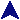Parallel Integration MasterSome PVM Examples```#include <stdio.h>
#include <string.h>
#include <stdlib.h>
#include <math.h>
#include <pvm3.h>
#include "func.h"

/*
* Find the area to the tolerance.
*/
static double find(double x1, double y1, double x2, double y2, double tol)
{
/* Compute the midpoint from the funcion. */
double midx = (x1 + x2) / 2;
double midy = f(midx);

/* Estimate the midpoint from the y value. */
double midest = (y1 + y2) / 2;

/* See if we're getting close. */
if(fabs(midy - midest) <= tol)
/* Will do.  Compute the area using the midy, since we
found it.  Two trapazoids algebraically simplified. */
return 0.25*(x2-x1)*(y1 + y2 + 2*midy);
else
/* Subdivide and try again. */
return find(x1, y1, midx, midy, tol) +
find(midx, midy, x2, y2, tol);
}

#define INITMAX 20

int main(int argc, char **argv)
{
int cc, tid;

/* Fine argument whine. */
if(argc < 5) {
printf("Usage: %s startx endx tol nreg funcargs*\n",
argv);
exit(2);
}

/* Get the args. */
char *pname = argv;
double start = atof(argv);
double end = atof(argv);
double tol = atof(argv);
int nreg = atoi(argv);

argc -= 5; argv += 5;

if(argc > INITMAX) {
printf("Function init limited to %d args.\n", INITMAX);
exit(4);
}

/* Sanity. */
if(tol < 0.0000000001) {
printf("Tolerance to too small or negative.\n");
exit(3);
}
if(nreg < 1) {
printf("Number of regions is nonpositive.\n");
exit(4);
}
if(end < start) {
double t = start;
start = end;
end = t;
}

int tids[nreg - 1];
if(nreg > 1) {
char buf;
strcat(strcpy(buf, pname), "_slave");
cc = pvm_spawn(buf, 0, 0, "", nreg - 1, tids);
if(cc <= 0) {
/* Nothing was spawned.  Whine about it. */
pvm_perror("Spawn failed");
pvm_exit();
exit(5);
}

if(cc != nreg - 1) {
/* At least one spawn failed.  Reduce the number
of regions to what we could get. */
nreg = cc + 1;
printf("Unable to spawn all slaves.  Reducing "
"to %d regions.\n", nreg);
}

/* Send out the function init information. */
int n;
for(n = 0; n < argc; n++) {
int len = strlen(argv[n]);
pvm_pkint(&len, 1, 1);
pvm_pkstr(argv[n]);
}
pvm_mcast(tids, nreg - 1, 1);
}

/* Initialize the function. */
finit(argc, argv);

/* Divide into to nreg regions and send all but one of
them to each of the slaves. */
double x = start;
double inc = (end - start) / nreg;
int i;
for(i = 0; i < nreg - 1; ++i) {
double newx = x + inc;
pvm_packf("%+ %lf %lf %lf ",
PvmDataDefault, x, newx, tol);
pvm_send(tids[i], 2);
x = newx;
}

/* Take the last region ourselves. */
double tot = find(x, f(x), end, f(end), tol);

/* Collect the others. */
for(i = 0; i < nreg - 1; ++i) {
int rn = pvm_recv(-1, 3);
double portion;
pvm_upkdouble(&portion, 1, 1);
tot += portion;
}

printf("Integral from %g to %g = %g (tol %g)\n",
start, end, tot, tol);

pvm_exit();
exit(0);
}

```